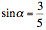Te Kete Ipurangi
Communities
Schools

### Te Kete Ipurangi user options:

Achievement objectives

What has changed:

# Achievement objective M8-6

In a range of meaningful contexts, students will be engaged in thinking mathematically and statistically. They will solve problems and model situations that require them to:

• manipulate trigonometric expressions.

## Indicators

• Solves problems that involve manipulating trig expressions using a variety of trig identities, including:
• the Pythagorean identities
• compound angle formula
• double angle formula
• sums and products
• reciprocal trigonometric functions.
• Identifies and uses appropriate key features of trigonometric functions, that is, amplitude, period and frequency.
• Makes links with solving equations M8-7, displaying and integrating graphs M8-2, and gradient functions M8-11.
• See key mathematical ideas on NZmaths.

## Possible context elaborations

• Proving identities, for example, double angle, half angle, triple angle formulae.
• Finding exact values of trig expressions, e.g. sin 105° and special triangles.
• Examples include: Given,, find the exact value of sin(α + β).
• Using trigonometry to solve problems involving triangles in 2-d or 3-d situations.
• Using the relationship between degree and radian measure to apply appropriate trig models to a given context.
• Solving trigonometric equations, giving general solutions or solutions within a given domain.
• Modelling situations such as tides using trigonometric functions.
• Activity: Big Bang Theory - Exact values

## Assessment for qualifications

NCEA achievement standards at levels 1, 2 and 3 have been aligned to the New Zealand Curriculum. Please ensure that you are using the correct version of the standards by going to the NZQA website.

The NZQA subject-specific resources pages are very helpful. From there, you can find all the achievement standards and links to assessment resources, both internal and external.

The following achievement standard(s) could assess learning outcomes from this AO:

Last updated September 14, 2018